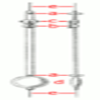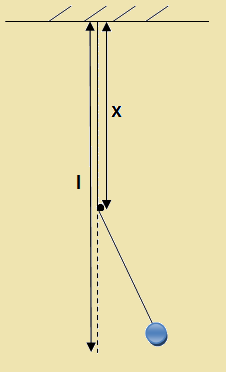#### You may also like### The Not-so-simple Pendulum 1

See how the motion of the simple pendulum is not-so-simple after all.### The Not-so-simple Pendulum 2

Things are roughened up and friction is now added to the approximate simple pendulum### Simple Pendulum

Get to grips with the variables behind the motion of a simple pendulum

##### Age 16 to 18 Challenge Level:

A time for one complete oscillation is a period $T$.  The period for a simple pendulum is

given by $T = 2\pi\sqrt{\frac{l}{g}}$ where $l$ is the length of the pendulum and $g$ is the acceleration of gravity.

1) Calculate the period for a simple pendulum if $l = 30$ cm and $g = 9.81 m/s^2$.

This period is not equal to 1 second. There are few possibilities to modify the pendulum or change the conditions to get the pendulum with the period of 1 second.2) First of all you can change the length of the pendulum. Find the required length.

3) It is possible to change the acceleration of gravity $g$ for example if you are in a lift which is accelerating. Find a new $g'$ if $l = 30$ cm as before. Is the lift moving up or down?

4) There is a bit more interesting way of modifying the pendulum.

Hammer a nail to the wall or make any other point obstacle for the thread and then swing the pendulum near the wall. Could you derive the period formula for this pendulum? Find $x$ in order to have the resultant period 1 second if $l = 30$cm and $g = 9.81 m/s^2$.

5) Could you think of any other way how to modify the pendulum?